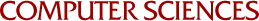## Minimum Error Bounds for Multidimensional Spline Approximation

J.B. Rosen
1970

Approximation of a smooth function f on a rectangular domain 9 c E' , by a tensor product of splines of degree m is considered. A basis for the product spline is formed using a single one-dimensional spline function. The approximation is computed, using linear programming, so as to minimize the maximum error on a discrete grid Q i 0, with grid size h. Realistic a posteriori bounds on the error in the uniform norm are given. Convergence of the approximation to a best approximation as h -+ 0 is shown. The extension to linear boundary value problems is also discussed.